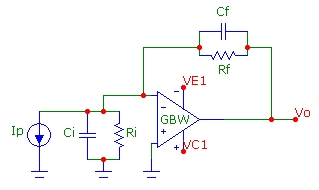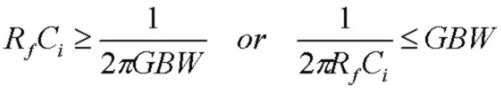# Transimpedance Circuit Output Noise-Voltage Calculator

Aug 26, 2012

This output noise voltage calculator applies to the transimpedance circuit shown below. Parameter names are described below the calculator:

Transimpedance Total Noise Calculator
GBW (MHz):      en (nV/√Hz):      in (pA/√Hz):
Rf (k):      Ri (k):      Cf (pF):      Ci (pF):
Resp(A/W):      Id(nA):      Psig (µW):
Q:   Fz (Hz):     Fp (Hz):   F0 (Hz):
f3db (Hz):   NBWen (Hz):   NBWin_th (Hz):
Ven (µV):   Vth (µV):   Vin(µV):
Vtotal(µV):   VId(µV):   VPsig(µV):

Transimpedance Cumulative Noise Calculator
fmax (MHz):      xm:   F0:
I1(xm,Q):   I2(xm,Q):
VenC(µV):   VthC(µV):   VinC(µV):
VtotalC(µV):   VIdC(µV):   VPsigC(µV):## Calculating The Noise With The Calculator

The calculator above can be used to compute the total (integrated over all frequency) and the cumulative (up to frequency fmax) output noise voltage. The noise sources include op-amp voltage and current noise sources, thermal noise sources and photodiode signal current and dark current shot noise sources. The calculation for both the total and cumulative noise integrates over the exact transfer function of the circuit, thereby taking into account the second-order nature of the response.
The steps are:
• Enter the circuit data in the first section (note units) and click Get Params & Noise which shows each noise component and the total along with other parameters
• S/N is the signal to noise ratio for the specified optical power Psig
• In the lower section, enter the fmax value (in MHz) to determine the cumulative output voltage noise up to this frequency
• Click Get Cumulative Noise which uses ALL the data in the upper section of the calculator
• The parameters xm, F0, I1(xm) and I2(xm) and the cumulative noise components and total cumulative noise up to fmax are calculated and displayed
Note that clicking Get Params & Noise does NOT update the data in the Cumulative Noise section of the calculator. If only TOTAL noise up to infinite frequency is required, the upper section of the calculator can be used independently of the lower section.
For convenience, 3 special buttons are provided which automatically calculate the required exact Cf value (given GBW, Rf, Ri and Ci) and all other parameters in the upper section of the calculator for the 3 useful cases of:
• Q = 0.50 the critically damped case; no peaking in frequency response and no overshoot in pulse response
• Q = 1/√2 the maximally flat case; maximally flat frequency repsonse with 4% overshoot in pulse response
• Q = 1.00 the "45deg PM" case; 15% peaking in frequency response with 16% overshoot in pulse response
The calculator is also available separately and also with a minimal interface.

Note on Special Conditions:
Depending on the component values of Rf, Ci and GBW, a solution for Cf for underdamped responses, such as the maximally flat condition Q=1/√2 or the popular Q=1.0 case may not be possible. This may occur for small values of Rf or Ci or GBW. (However, a Cf solution for the critically damped case Q = 0.5 will always be possible). For the "maximally flat" case with Q = 1/√2, a Cf solution will only be possible under this condition:For the common case with Q = 1.0, a Cf solution will only be possible under this condition:In both cases, this means that the zero frequency of the noise gain (or equivalently the pole frequency of the feedback factor β(f)) must be comparable to or less the op-amp GBW for a Cf solutions with these Q values to exist. This condition will NOT be satisfied if the zero and pole frequencies of the noise-gain are considerably higher than the op-amp GBW. In that case, the transimpedance f3db bandwidth will simply be controlled by the op-amp open-loop rolloff and f3db will be comparable to the op-amp GBW. Under this condition, the circuit will typically have a Q value < 0.5 with Cf set to zero (or some nominally small stray value such as 0.3 pF) and there will be negligible noise-gain peaking in the pass-band of the transimpedance response. The circuit will have considerable phase margin and will be stable so that no compensation capacitance Cf will be required. Of course Cf could be added to reduce f3db and total output noise.

## Calculator Parameters

• GBW    op-amp gain-bandwidth product
• en    op-amp noise voltage spectral density
• in    op-amp noise current spectral density
• Rf    feedback resistance
• Ri    total shunt resistance at inverting-input to ground
• Cf    feedback shunt capacitance
• Ci    total capacitance at inverting-input to ground
• Resp    photodiode responsivity in A/W
• Id    photodiode dark current
• Psig    optical power received by photodiode

• Q    quality factor of transimpedance transfer function
• Fz    zero frequency of noise-gain function
• Fp    pole frequency of noise-gain function
• F0    natural resonant frequency of transimpedance transfer function
• f3db    3 dB bandwidth of transimpedance transfer function
• NBWen    effective "noise bandwidth" for en contribution
• NBWin_th     effective "noise bandwidth" for Rf thermal or in contribution

• Ven    total output noise voltage from en contribution
• Vth    total output noise voltage from Rf thermal contribution
• Vin    total output noise voltage from in contribution
• VId    total output noise voltage from photodiode dark current Id contribution
• VPsig    total output noise voltage from photocurrent signal shot noise contribution
• Vtotal    total output noise voltage of all contributions (rms summed)
• S/N    signal to noise ratio (at optical power level Psig) in dB

• fmax    maximum frequency for cumulative noise voltage
• xm    parameter for I1, I2 integrals xm = fmax/F0
• I1(xm,Q), I2(xm,Q)     cumulative noise integrals
• VenC    cumulative output noise voltage from en contribution up to fmax
• VthC    cumulative output noise voltage from Rf thermal contribution up to fmax
• VinC    cumulative output noise voltage from in contribution up to fmax
• VIdC    cumulative output noise voltage from photodiode dark current Id contribution up to fmax
• VPsigC    cumulative output noise voltage from photocurrent shot noise contribution up to fmax
• VtotalC    cumulative output noise voltage of all contributions (rms summed) up to fmax